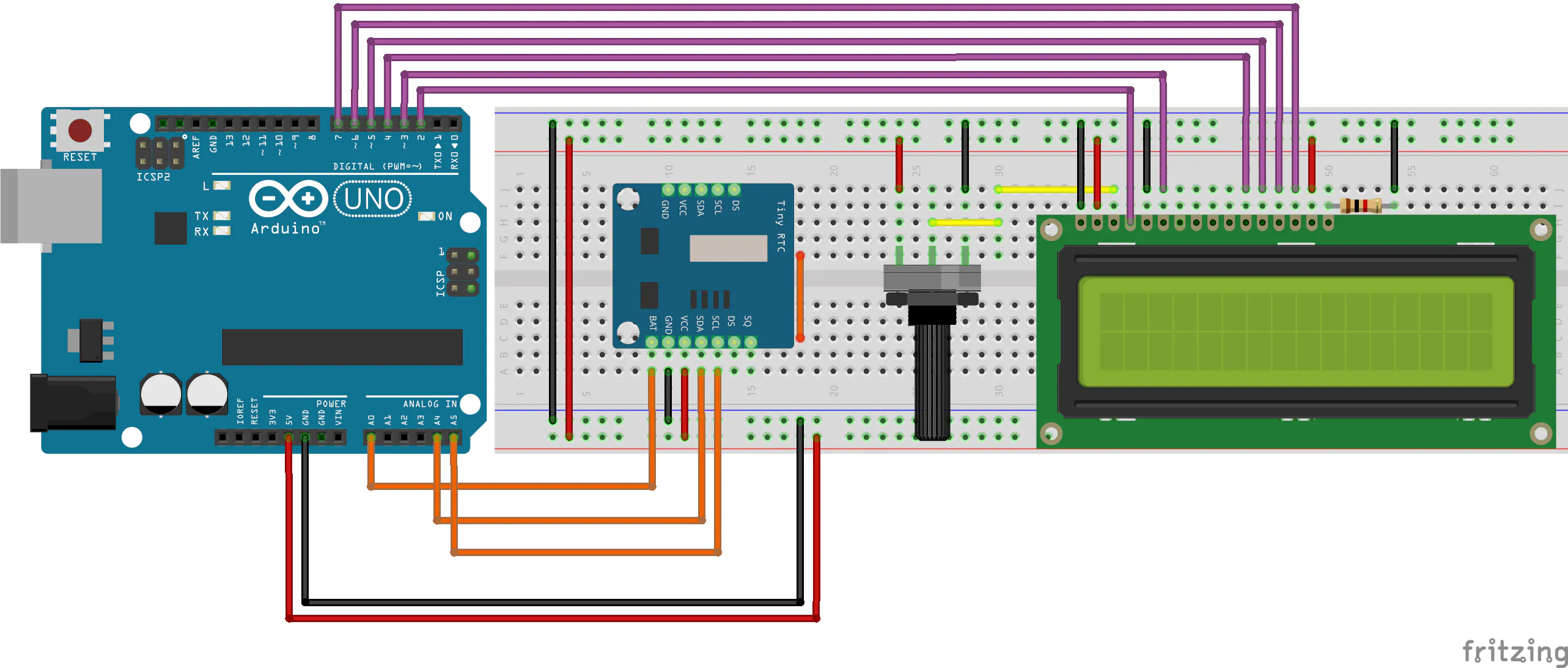# 需要的元件

• Arduino UNO• LCD 1602

• Arduino Tiny RTC I2C模块 24C32存储器 DS1307时钟

• Arduino IDE# 原理及流程

### 项目发展#include <LiquidCrystal.h>
#include <Wire.h>
#include "RTClib.h"

RTC_DS1307 rtc;

const int rs = 2, en = 3, d4 = 4, d5 = 5, d6 = 6, d7 = 7;
LiquidCrystal lcd(rs, en, d4, d5, d6, d7);

#define tensao A0

bool ControlVbat1 = 0, ControlVbat2 = 0;

void setup ()
{
lcd.begin(16,2);
Serial.begin(9600);
if (! rtc.begin())
{
while(1);
}
if (! rtc.isrunning())
{
Serial.println("DS1307 rodando!");
rtc.adjust(DateTime(2025, 6, 1, 15, 00, 00)); //Year, Month, Day, Minutes and Seconds
}
pinMode(tensao, INPUT);
}

void loop ()
{

DateTime now = rtc.now();
lcd.setCursor(0,0);

if(now.hour() < 10)
{
lcd.setCursor(0,0);
lcd.print("0");
}

lcd.print(now.hour(), DEC );
lcd.print(":");

if(now.minute() < 10)
{
lcd.setCursor(3,0);
lcd.print("0");
}

lcd.print(now.minute(), DEC);
lcd.setCursor(8,0);
lcd.print(now.day(), DEC );
lcd.print("/");
lcd.print(now.month(), DEC);
lcd.print("/");
lcd.print(now.year(), DEC);
if(Vbat < 1.5 && ControlVbat1 == 0)
{
lcd.setCursor(0,1);
lcd.print("                ");
lcd.setCursor(2,1);
lcd.print("Low Battery!");
ControlVbat1 = 1;
ControlVbat2 = 0;
}
if(Vbat >= 1.5 && ControlVbat2 == 0)
{
lcd.setCursor(0,1);
lcd.print("                ");
lcd.setCursor(1,1);
lcd.print("Voltage: ");
lcd.print(Vbat);
lcd.print("V");
ControlVbat1 = 0;
ControlVbat2 = 1;
}
}


float Vbat = (5*(analogRead(tensao)))/1023;


DateTime now = rtc.now();
lcd.setCursor(0,0);

if(now.hour() < 10)
{
lcd.setCursor(0,0);
lcd.print("0");
}

lcd.print(now.hour(), DEC );
lcd.print(":");

if(now.minute() < 10)
{
lcd.setCursor(3,0);
lcd.print("0");
}

lcd.print(now.minute(), DEC);
lcd.setCursor(8,0);
lcd.print(now.day(), DEC );
lcd.print("/");
lcd.print(now.month(), DEC);
lcd.print("/");
lcd.print(now.year(), DEC);


if(Vbat < 1.5 && ControlVbat1 == 0)
{
lcd.setCursor(0,1);
lcd.print("                ");
lcd.setCursor(2,1);
lcd.print("Low Battery!");
ControlVbat1 = 1;
ControlVbat2 = 0;
}
if(Vbat >= 1.5 && ControlVbat2 == 0)
{
lcd.setCursor(0,1);
lcd.print("                ");
lcd.setCursor(1,1);
lcd.print("Voltage: ");
lcd.print(Vbat);
lcd.print("V");
ControlVbat1 = 0;
ControlVbat2 = 1;
}LCD显示电量不足消息。# 最后0/250

arduino

### 加入组织